## Stay Hungry.Stay Foolish.

Reinforcement Learning 的核心基础概念及实现

2013 年伦敦的一家小公司 DeepMind 发表了一篇论文 Playing Atari with Deep Reinforcement Learning 。论文描述了如何教会电脑玩 Atari 2600 游戏（仅仅让电脑观察游戏的每一帧图像和接受游戏分数的上升作为奖励信号）。结果很令人满意，因为电脑比大多数人类玩家玩的好，而且该模型在没有任何改变的情况下，学会了玩其他游戏，并且在三个游戏中表现比人类玩家好！自此通用人工智能的话题开始火热 -- 能够适应各种负责环境而不仅仅局限于玩棋类游戏，而 DeepMind 因此被谷歌看中而被收购。2015 年，DeepMind 又发表了一篇 Human-level control through deep reinforcement learning，在本篇论文中 DeepMind 用同样的模型，教会电脑玩49种游戏，而且过半游戏比专业玩家玩得更好。2016年3月，AlphaGo 与围棋世界冠军、职业九段选手李世石进行人机大战，并以4:1的总比分获胜；2016年末2017年初，该程序在中国棋类网站上以“大师”（Master）为注册帐号与中日韩数十位围棋高手进行快棋对决，连续60局无一败绩。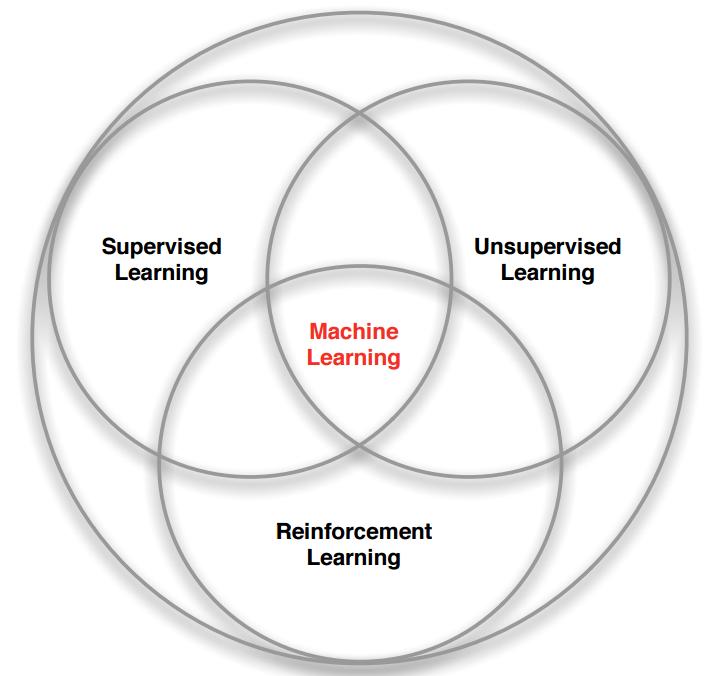## 强化学习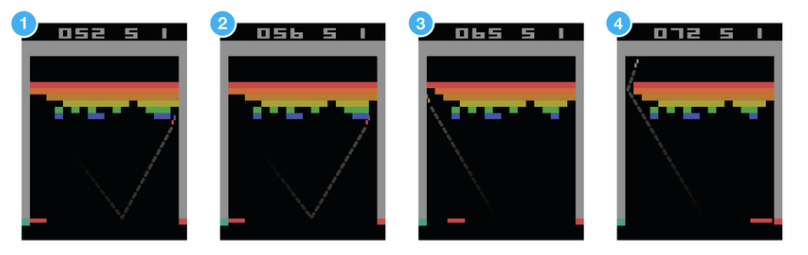## 马尔可夫决策过程 （Markov Decision Process）

MDP 中有两个对象：AgentEnvironment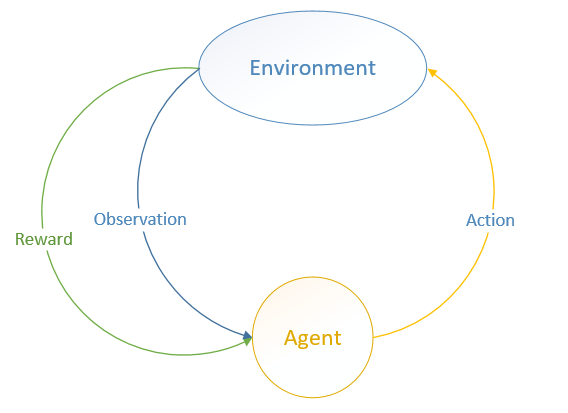Environment 处于一个特定的状态State）（如打砖块游戏中挡板的位置、各个砖块的状态等），Agent 可以通过执行特定的动作Actions ）（如向左向右移动挡板）来改变 Environment状态Environment 状态改变之后会返回一个观察Observation）给Agent，同时还会得到一个奖励Reward）（可以为负，就是惩罚），这样 Agent 根据返回的信息采取新的动作，如此反复下去。Agent 如何选择动作叫做策略Policy）。MDP 的任务就是找到一个策略，来最大化奖励。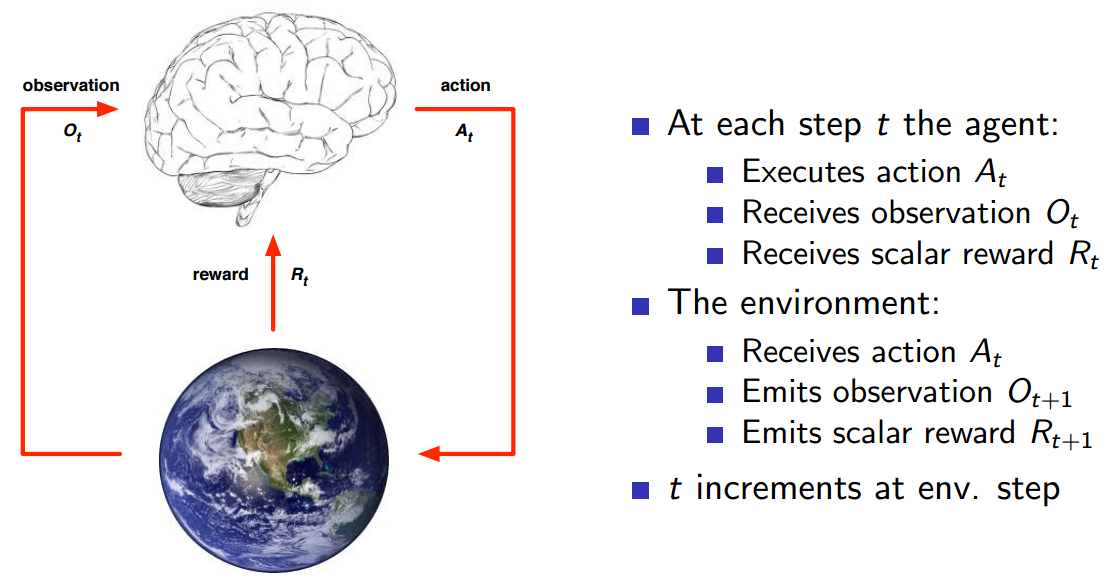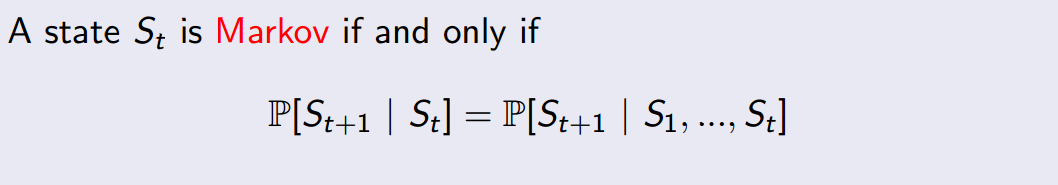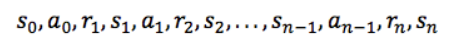## 折扣未来奖励（Discounted Future Reward）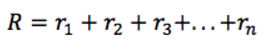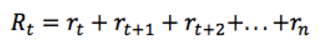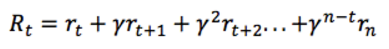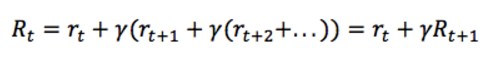## Q-learning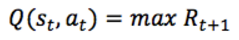Q(s，a) 看作是在状态 s，执行动作 a时，游戏结束时的分数就行了。不要惊奇为什么在状态 s 就可以知道游戏结束时的分数，因为这是马尔科夫过程，将来只和现在有关，现在是将来的充分条件。这样最大化 Q(s，a) 就相当于最大化我们游戏的得分了（至于为什么叫做 Q，因为它代表了在特定状态 s 下特定动作 a 的质量"quality"）。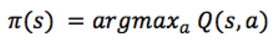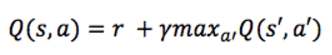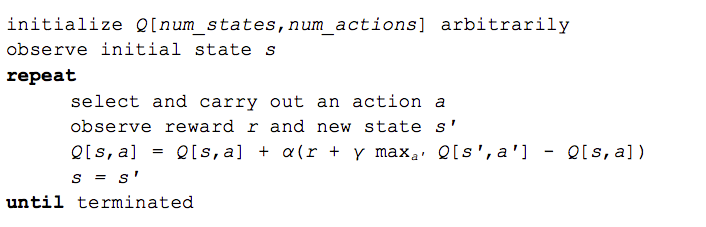α是一个学习速率（learning rate），它控制了先前的Q值和新的Q值之间的差异有多少被考虑在内。 特别地，当α = 1时，则两个 Q [s，a] 抵消，更新与贝尔曼方程完全相同。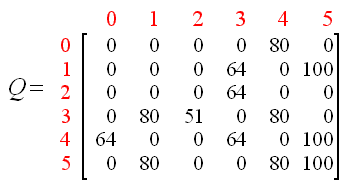## Deep Q Network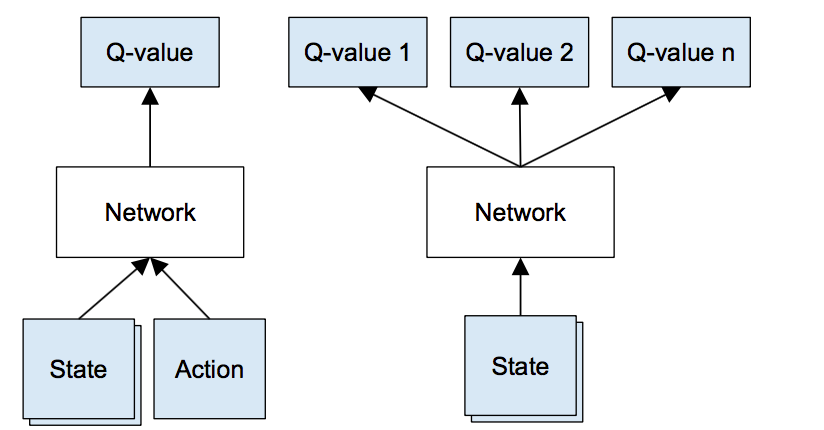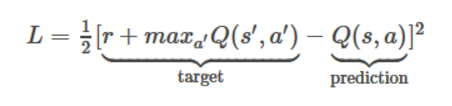1. 对当前状态 s 进行前向传播以获取所有动作的Q值。
2. 对下一个状态 s' 进行前向传播，并计算最大Q值 max Q(s’, a’)
3. 将动作对应的目标Q值设置为 r + γ max Q（s'，a'）（步骤2中计算的最大值）。
4. 使用反向传播算法更新参数。

## 探索与开发（Exploration-Exploitation）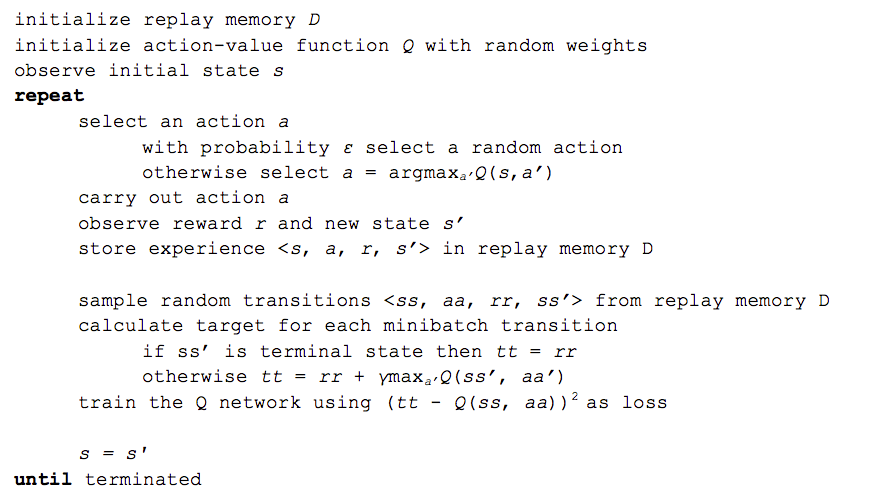DeepMind 还有更多的技巧来优化，如目标网络，错误剪辑，奖励剪辑等，但这些都不在此介绍范围之内。

## 实现

Q 算法的 Q函数我么可以用任何函数来代替，不限于神经网络或者卷积神经网络，因此我们需要把它抽象出来：

class Model():
def __init__(self, num_outputs):
self.num_outputs = num_outputs

def definition(self):
raise NotImplementedError

def get_num_outputs(self):
return self.num_outputs

Model 代表了Q函数的抽象，num_outputs 代表了可能的动作个数，CartPole 中有两个：左移或者右移；FlappyBird 也有两个动作：点击屏幕或者什么都不做。definition 函数代表具体的Q函数定义，返回输入和输出。

# Env-related abstractions
class Env():
def step(self, action_index):
raise NotImplementedError

def reset(self):
raise NotImplementedError

def render(self):
raise NotImplementedError

Env 可以是任何游戏，或者其他问题。step 函数表示执行指定动作 action_index，然后返回一个元组：(state, reward, terminal, info)state 是游戏当前状态，如屏幕像素；reward 是奖励，可以为负数； terminal 代表游戏是否结束；info 代表一些其他信息，可有可无。

import numpy as np
import tensorflow as tf
import random
import numpy as np
import time
import sys
import collections

class DeepQNetwork():
def __init__(self,
model,
env,
learning_rate=0.001,
gamma=0.9,
replay_memeory_size=10000,
batch_size=32,
initial_epsilon=0.5,
final_epsilon=0.01,
decay_factor=1,
logdir=None,
save_per_step=1000,
test_per_epoch=100):
self.model = model
self.env = env
self.num_actions = model.get_num_outputs()
self.learning_rate = learning_rate
self.optimizer = optimizer
self.gamma = gamma
self.epsilon = initial_epsilon
self.initial_epsilon = initial_epsilon
self.final_epsilon = final_epsilon
self.decay_factor = decay_factor
self.logdir = logdir
self.test_per_epoch = test_per_epoch

self.replay_memeory = collections.deque()
self.replay_memeory_size = replay_memeory_size
self.batch_size = batch_size
self.define_q_network()
# session
self.sess = tf.InteractiveSession()
self.sess.run(tf.global_variables_initializer())
if self.logdir is not None:
if not self.logdir.endswith('/'): self.logdir += '/'
self.save_per_step = save_per_step
self.saver = tf.train.Saver()
checkpoint_state = tf.train.get_checkpoint_state(self.logdir)
if checkpoint_state and checkpoint_state.model_checkpoint_path:
path = checkpoint_state.model_checkpoint_path
self.saver.restore(self.sess, path)
print('Restore from {} successfully.'.format(path))
else:
print('No checkpoint.')
self.summaries = tf.summary.merge_all()
self.summary_writer = tf.summary.FileWriter(
self.logdir, self.sess.graph)
sys.stdout.flush()

def define_q_network(self):
self.input_states, self.q_values = self.model.definition()
self.input_actions = tf.placeholder(tf.float32,
[None, self.num_actions])
# placeholder of target q values
self.input_q_values = tf.placeholder(tf.float32, [None])
# only use selected q values
action_q_values = tf.reduce_sum(
tf.multiply(self.q_values, self.input_actions),
reduction_indices=1)

self.global_step = tf.Variable(0, trainable=False)
# define cost
self.cost = tf.reduce_mean(
tf.square(self.input_q_values - action_q_values))
self.optimizer = self.optimizer(self.learning_rate).minimize(
self.cost, global_step=self.global_step)
tf.summary.scalar('cost', self.cost)
tf.summary.scalar('reward', tf.reduce_mean(action_q_values))

def egreedy_action(self, state):
if random.random() <= self.epsilon:
action_index = random.randint(0, self.num_actions - 1)
else:
action_index = self.action(state)
if self.epsilon > self.final_epsilon:
self.epsilon *= self.decay_factor
return action_index

def action(self, state):
q_values = self.q_values.eval(feed_dict={self.input_states:
[state]})
return np.argmax(q_values)

def do_train(self, epoch):
# randomly select a batch
mini_batches = random.sample(self.replay_memeory, self.batch_size)
state_batch = [batch for batch in mini_batches]
action_batch = [batch for batch in mini_batches]
reward_batch = [batch for batch in mini_batches]
next_state_batch = [batch for batch in mini_batches]

# target q values
target_q_values = self.q_values.eval(
feed_dict={self.input_states: next_state_batch})
input_q_values = []
for i in range(len(mini_batches)):
terminal = mini_batches[i]
if terminal:
input_q_values.append(reward_batch[i])
else:
# Discounted Future Reward
input_q_values.append(reward_batch[i] +
self.gamma * np.max(target_q_values[i]))
feed_dict = {
self.input_actions: action_batch,
self.input_states: state_batch,
self.input_q_values: input_q_values
}
self.optimizer.run(feed_dict=feed_dict)
step = self.global_step.eval()
if self.saver is not None and epoch > 0 and step % self.save_per_step == 0:
summary = self.sess.run(self.summaries, feed_dict=feed_dict)
self.summary_writer.flush()
self.saver.save(self.sess, self.logdir + 'dqn', self.global_step)

# num_epoches: train epoches
def train(self, num_epoches):
for epoch in range(num_epoches):
epoch_rewards = 0
state = self.env.reset()
# 9999999999: max step per epoch
for step in range(9999999999):
# ε-greedy exploration
action_index = self.egreedy_action(state)
next_state, reward, terminal, info = self.env.step(
action_index)
# one-hot action
one_hot_action = np.zeros([self.num_actions])
one_hot_action[action_index] = 1
# store trans in replay_memeory
self.replay_memeory.append((state, one_hot_action, reward,
next_state, terminal))
# remove element if exceeds max size
if len(self.replay_memeory) > self.replay_memeory_size:
self.replay_memeory.popleft()

# now train the model
if len(self.replay_memeory) > self.batch_size:
self.do_train(epoch)

# state change to next state
state = next_state
epoch_rewards += reward
if terminal:
# Game over. One epoch ended.
break
# print("Epoch {} reward: {}, epsilon: {}".format(
#     epoch, epoch_rewards, self.epsilon))
# sys.stdout.flush()

#evaluate model
if epoch > 0 and epoch % self.test_per_epoch == 0:
self.test(epoch, max_step_per_test=99999999)

def test(self, epoch, num_testes=10, max_step_per_test=300):
total_rewards = 0
print('Testing...')
sys.stdout.flush()
for _ in range(num_testes):
state = self.env.reset()
for step in range(max_step_per_test):
# self.env.render()
action = self.action(state)
state, reward, terminal, info = self.env.step(action)
total_rewards += reward
if terminal:
break
average_reward = total_rewards / num_testes
print("epoch {:5} average_reward: {}".format(epoch, average_reward))
sys.stdout.flush()

__init__ 方法除了 envmodel 是必须的，其他都是可选的。

1. optimizer 指定了 Tensorflow 使用的优化器；
2. learning_rate 是学习速率；
3. gamma 就是算法中的折扣因子 γ
4. replay_memeory_size 是存放经验的最大数量；
5. batch_size 是从经验池取经验用于训练的大小；
6. epsilon 就是 ε-greedy exploration 中的 ε，表示 epsilon 将从 initial_epsilondecay_factor 的速率下降到 final_epsilon 为止，decay_factor 等于1表示不下降；
7. logdir 是 Tensorflow 保存模型的路径，为 None 表示不保存训练结果；
8. save_per_step 是每多少步保存一下断点；
9. test_per_epoch 是每多少不测试评估一下训练进度。

def define_q_network(self):
self.input_states, self.q_values = self.model.definition()
self.input_actions = tf.placeholder(tf.float32,
[None, self.num_actions])
# placeholder of target q values
self.input_q_values = tf.placeholder(tf.float32, [None])
# only use selected q values
action_q_values = tf.reduce_sum(
tf.multiply(self.q_values, self.input_actions),
reduction_indices=1)

self.global_step = tf.Variable(0, trainable=False)
# define cost
self.cost = tf.reduce_mean(
tf.square(self.input_q_values - action_q_values))
self.optimizer = self.optimizer(self.learning_rate).minimize(
self.cost, global_step=self.global_step)
tf.summary.scalar('cost', self.cost)
tf.summary.scalar('reward', tf.reduce_mean(action_q_values))

# num_epoches: train epoches
def train(self, num_epoches):
for epoch in range(num_epoches):
epoch_rewards = 0
state = self.env.reset()
# 9999999999: max step per epoch
for step in range(9999999999):
# ε-greedy exploration
action_index = self.egreedy_action(state)
next_state, reward, terminal, info = self.env.step(
action_index)
# one-hot action
one_hot_action = np.zeros([self.num_actions])
one_hot_action[action_index] = 1
# store trans in replay_memeory
self.replay_memeory.append((state, one_hot_action, reward,
next_state, terminal))
# remove element if exceeds max size
if len(self.replay_memeory) > self.replay_memeory_size:
self.replay_memeory.popleft()

# now train the model
if len(self.replay_memeory) > self.batch_size:
self.do_train(epoch)

# state change to next state
state = next_state
epoch_rewards += reward
if terminal:
# Game over. One epoch ended.
break
# print("Epoch {} reward: {}, epsilon: {}".format(
#     epoch, epoch_rewards, self.epsilon))
# sys.stdout.flush()

#evaluate model
if epoch > 0 and epoch % self.test_per_epoch == 0:
self.test(epoch, max_step_per_test=99999999)

def do_train(self, epoch):
# randomly select a batch
mini_batches = random.sample(self.replay_memeory, self.batch_size)
state_batch = [batch for batch in mini_batches]
action_batch = [batch for batch in mini_batches]
reward_batch = [batch for batch in mini_batches]
next_state_batch = [batch for batch in mini_batches]

# target q values
target_q_values = self.q_values.eval(
feed_dict={self.input_states: next_state_batch})
input_q_values = []
for i in range(len(mini_batches)):
terminal = mini_batches[i]
if terminal:
input_q_values.append(reward_batch[i])
else:
# Discounted Future Reward
input_q_values.append(reward_batch[i] +
self.gamma * np.max(target_q_values[i]))
feed_dict = {
self.input_actions: action_batch,
self.input_states: state_batch,
self.input_q_values: input_q_values
}
self.optimizer.run(feed_dict=feed_dict)
step = self.global_step.eval()
if self.saver is not None and epoch > 0 and step % self.save_per_step == 0:
summary = self.sess.run(self.summaries, feed_dict=feed_dict)
self.summary_writer.flush()
self.saver.save(self.sess, self.logdir + 'dqn', self.global_step)

### CartPole

CartPole 比较简单，状态只是一个一个4维的实数值向量，像这样：

[-0.00042486 -0.02710707  0.01032103  -0.04882064]

class CartPoleEnv(Env):
def __init__(self):
self.env = gym.make('CartPole-v0')

def step(self, action_index):
s, r, t, i = self.env.step(action_index)
return s, r, t, i

def reset(self):
return self.env.reset()

def render(self):
self.env.render()

model = SimpleNeuralNetwork([4, 16, 2])

model = SimpleNeuralNetwork([4, 16, 2])
env = CartPoleEnv()
qnetwork = DeepQNetwork(
model=model, env=env, learning_rate=0.0001, logdir='./tmp/CartPole/')
qnetwork.train(4000)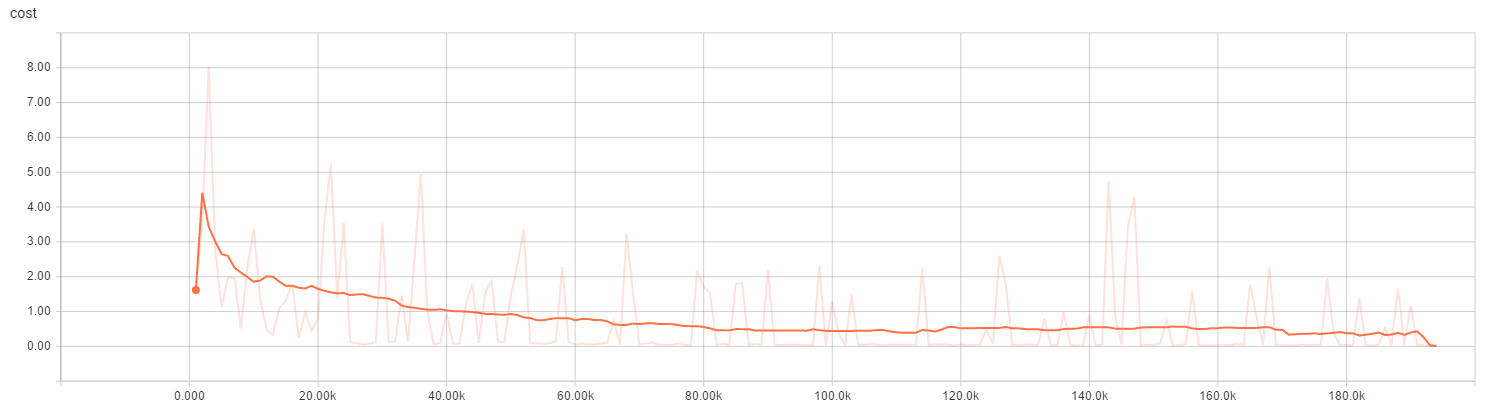CartPole损失变化曲线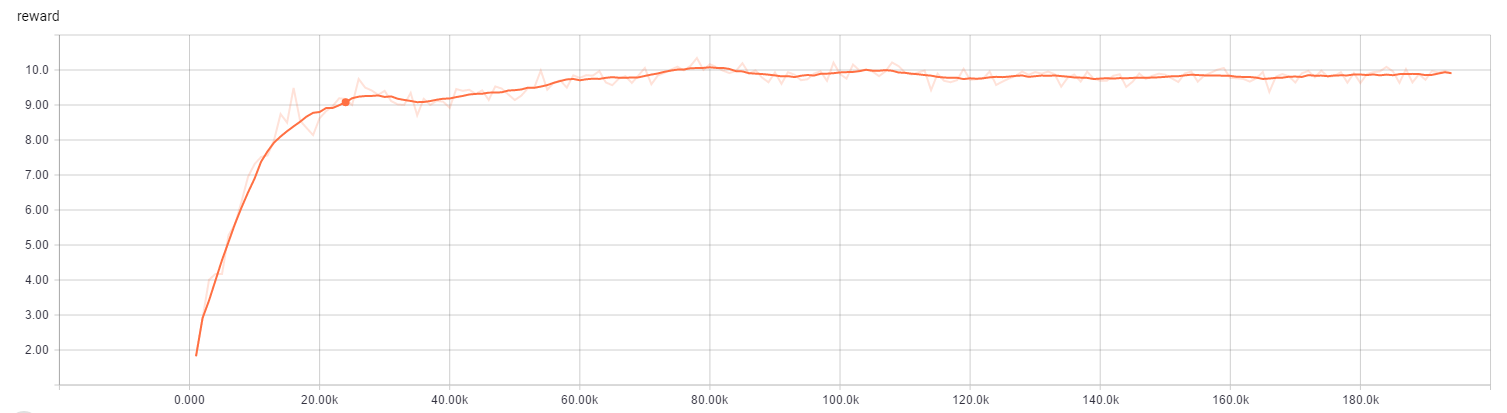CartPole奖励变化曲线

### FlappyBird

FlappyBird 比较复杂，我们采用原始屏幕像素作为输入。但是为了加快收敛，使用了一下技巧：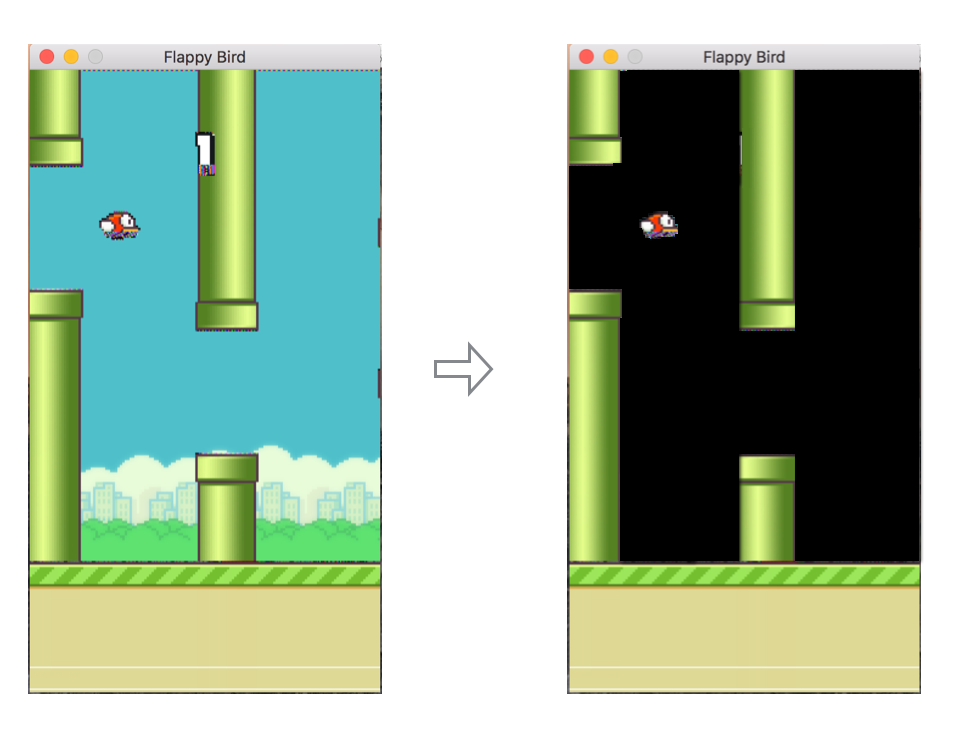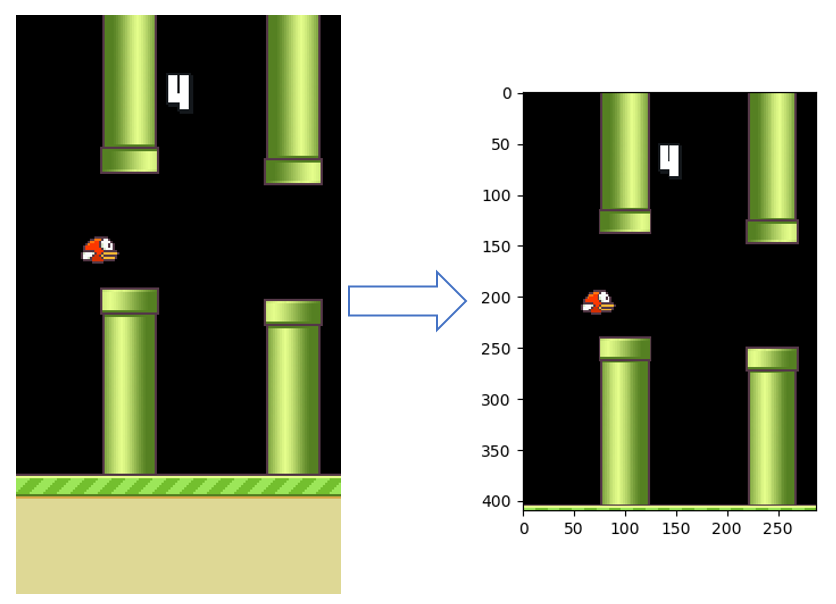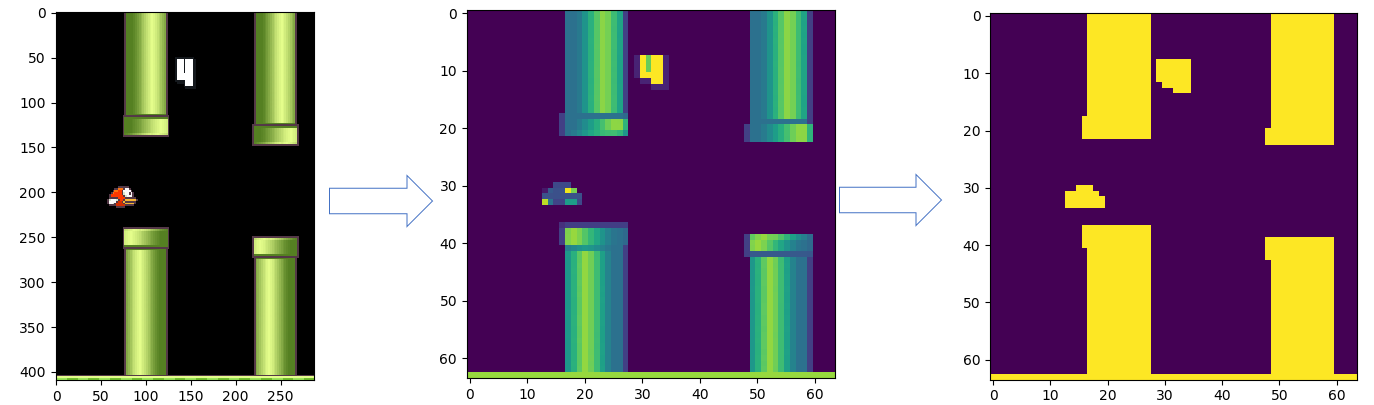model = CNN(img_w=image_size, img_h=image_size, num_outputs=2)
env = FlappyBirdEnv()
qnetwork = DeepQNetwork(
model=model,
env=env,
learning_rate=1e-8,
initial_epsilon=0.1,
final_epsilon=0,
decay_factor=0.99,
save_per_step=1000,
logdir='./tmp/FlappyBird/')
if train:
qnetwork.train(10000)
else:
runGame(env, network)

def egreedy_action(self, state):
#Exploration
if random.random() <= self.epsilon:
if random.random() < 0.95:
action_index = 0
else:
action_index = 1
# action_index = random.randint(0, self.num_actions - 1)
else:
#Exploitation
action_index = self.action(state)
if self.epsilon > self.final_epsilon:
self.epsilon *= self.decay_factor
return action_index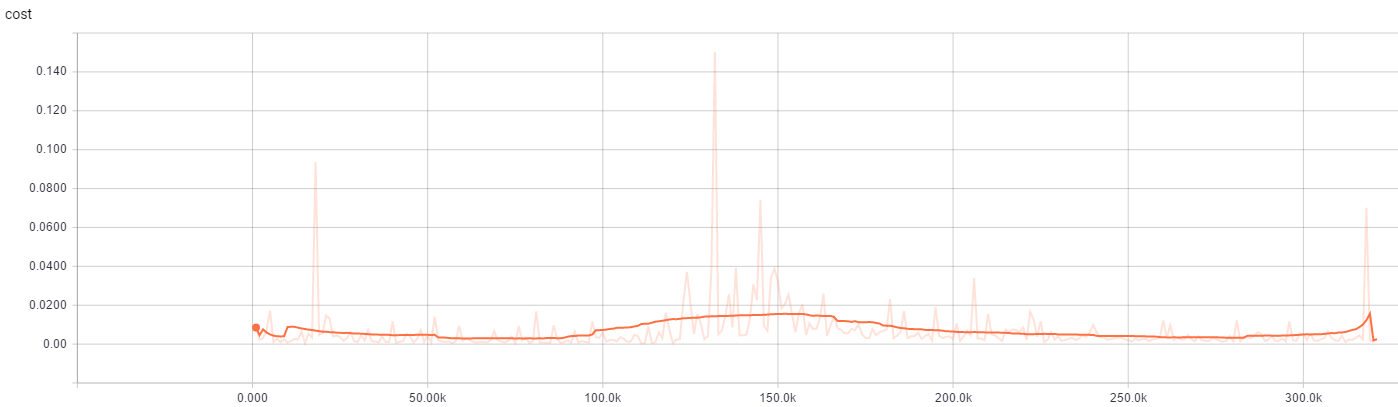FlappyBird损失变化曲线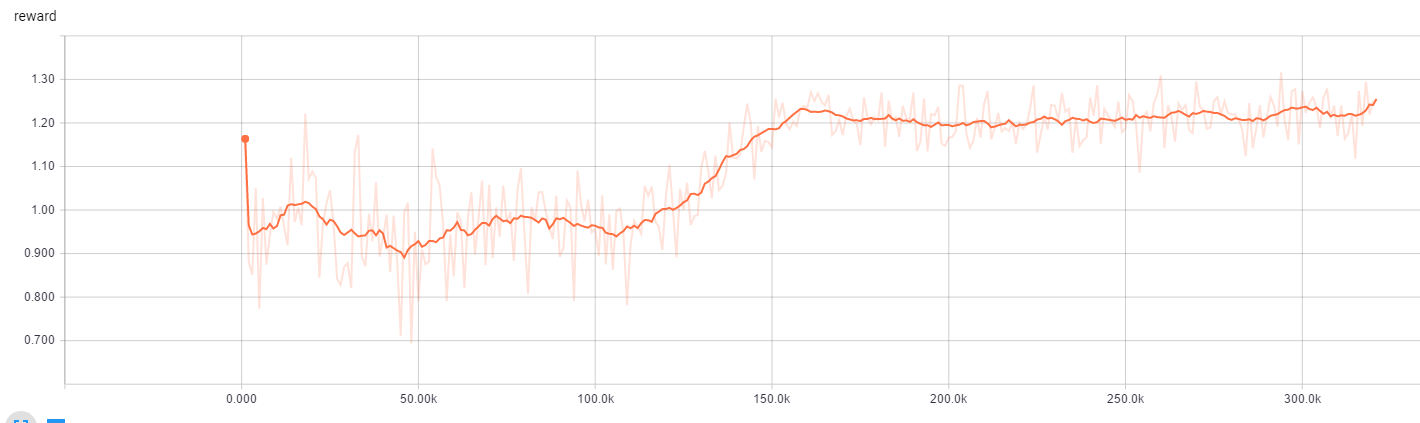FlappyBird奖励变化曲线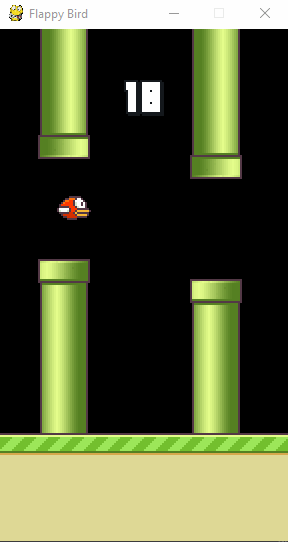## 参考

⬆️

2017-06-15 17:20

2017-07-23 00:48

haolihai

lufficc
2017-08-03 20:37

@jimmy 需要把 disk 修改为 local

2017-08-11 09:56

bream